1. /
2. CBSE
3. /
4. Class 06
5. /
6. Mathematics
7. /
8. NCERT Solutions for Class...

# NCERT Solutions for Class 6 Maths Exercise 4.1

NCERT solutions for Maths Basic geometrical ideas## NCERT Solutions for Class 6 Maths Basic geometrical ideas

###### Question 1.Use the figure to name: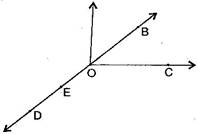(a)Five points

(b)A line

(c)Four rays

(d)Five line segments

(a) Five points are: O, B, C, D, E

(b) A line: {tex}\overline {{\text{DE}}} ,{/tex}{tex}\overline {{\text{DB}}} ,{/tex}{tex}\overline {{\text{OE}}} ,{/tex}{tex}\overline {{\text{OB}}} {/tex}

(c) Four rays: {tex}\overrightarrow {{\text{OD}}} ,{/tex}{tex}\overrightarrow {{\text{OE}}} ,{/tex}{tex}\overrightarrow {{\text{OC}}} ,{/tex}{tex}\overrightarrow {{\text{OB}}} {/tex}

(d) Four line segments: {tex}\overline {{\text{DE}}} ,{/tex}{tex}\overline {{\text{OE}}} ,{/tex}{tex}\overline {{\text{OC}}} ,{/tex}{tex}\overline {{\text{OB}}} ,{/tex}, {tex}\overline {{\text{OD}}} {/tex}

NCERT Solutions for Class 6 Maths Exercise 4.1

###### Question 2.Name the line given in all possible (twelve) ways, choosing only two letters at a time from the four given.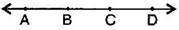Answer: {tex}\overline {{\text{AB}}} ,{/tex}{tex}\overline {{\text{AC}}} ,{/tex}{tex}\overline {{\text{AD}}} ,{/tex}{tex}\overline {{\text{BC}}} ,{/tex}{tex}\overline {{\text{BD}}} ,{/tex}{tex}\overline {{\text{CD}}} ,{/tex}{tex}\overline {{\text{BA}}} ,{/tex}{tex}\overline {{\text{CA}}} ,{/tex}{tex}\overline {{\text{DA}}} ,{/tex}{tex}\overline {{\text{CB}}} ,{/tex}{tex}\overline {{\text{DB}}} ,{/tex}{tex}\overline {{\text{DC}}} {/tex}

###### Question 3.Use the figure to name: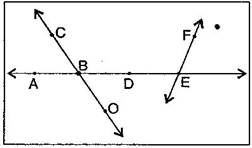(a)Line containing point E.

(b)Line passing through A.

(c)Line on which O lies.

(d)Two pairs of intersecting lines.

Answer: (a) A line containing E = {tex}\overline {{\text{AE}}} {/tex} or {tex}\overline {{\text{FE}}} {/tex}

(b) A line passing through A = {tex}\overline {{\text{AE}}} {/tex} or {tex}\overline {{\text{DE}}} {/tex}

(c) A line on which O lies = {tex}\overline {{\text{CO}}} {/tex} or {tex}\overline {{\text{OC}}} {/tex}

(d) Two pairs of intersecting lines are : {tex}\overline {{\text{AD}}} ,{/tex}{tex}\overline {{\text{CO}}} {/tex} and {tex}\overline {{\text{AE}}} {/tex}, {tex}\overline {{\text{FE}}} {/tex}

NCERT Solutions for Class 6 Maths Exercise 4.1

###### Question 4.How many lines can pass though:

(a) one given point?

(b) two given points

Answer: (a) Infinite number of lines can pass through one given point.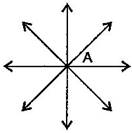(b) Only one line can pass through two given points.

###### Question 5.Draw a rough figure and label suitably in each of the following cases:

(a)Point P lies on {tex}\overline {{\text{AB}}} .{/tex}

(b) {tex}\overline {{\text{XY}}} {/tex} and {tex}\overline {{\text{PQ}}} {/tex} intersect at M.

(c) Line {tex}l{/tex} contains E and F but not D.

(d) {tex}\overline {{\text{OP}}} {/tex} and {tex}\overline {{\text{OQ}}} {/tex} meet at O.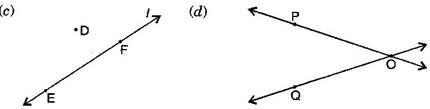NCERT Solutions for Class 6 Maths Exercise 4.1

###### Question 6.Consider the following figure of line {tex}\overline {{\text{MN}}} {/tex}. Say whether following statements are true or false in the context of the given figure: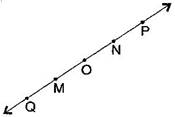(a)Q, M, O, N, P are points on the line {tex}\overline {{\text{MN}}} {/tex}.

(b)M, O, N are points on a line segment {tex}\overline {{\text{MN}}} {/tex}.

(c)M and N are end points of line segment {tex}\overline {{\text{MN}}} {/tex}.

(d)O and N are end points of line segment {tex}\overline {{\text{OP}}} {/tex}.

(e)M is one of the end points of line segment {tex}\overline {{\text{QO}}} {/tex}.

(f)M is point on ray {tex}\overrightarrow {{\text{OP}}} {/tex}.

(g)Ray {tex}\overrightarrow {{\text{OP}}} {/tex} is different from ray {tex}\overrightarrow {{\text{OP}}} {/tex}.

(h)Ray {tex}\overrightarrow {{\text{OP}}} {/tex}same as ray {tex}\overrightarrow {{\text{OM}}} .{/tex}.

(i)Ray {tex}\overrightarrow {{\text{OM}}} .{/tex} is not opposite to ray {tex}\overrightarrow {{\text{OP}}} {/tex}.

(j)O is not an initial point of {tex}\overline {{\text{NP}}} {/tex} and {tex}\overline {{\text{NM}}} {/tex}.

Answer: (a) True, (b) True, (c) True, (d) False, (e) False, (f) False, (g) True, (h) False, (i) False, (j) False, (k) True

## NCERT Solutions for Class 6 Maths Exercise 4.1

NCERT Solutions Class 6 Maths PDF (Download) Free from myCBSEguide app and myCBSEguide website. Ncert solution class 6 Maths includes text book solutions from Class 6 Maths Book . NCERT Solutions for CBSE Class 6 Maths have total 14 chapters. 6 Maths NCERT Solutions in PDF for free Download on our website. Ncert Maths class 6 solutions PDF and Maths ncert class 6 PDF solutions with latest modifications and as per the latest CBSE syllabus are only available in myCBSEguide.

## CBSE app for Students

To download NCERT Solutions for Class 6 Maths, Social Science Computer Science, Home Science, Hindi English, Maths Science do check myCBSEguide app or website. myCBSEguide provides sample papers with solution, test papers for chapter-wise practice, NCERT solutions, NCERT Exemplar solutions, quick revision notes for ready reference, CBSE guess papers and CBSE important question papers. Sample Paper all are made available through the best app for CBSE students and myCBSEguide website.Test Generator

Create question paper PDF and online tests with your own name & logo in minutes.myCBSEguide

Question Bank, Mock Tests, Exam Papers, NCERT Solutions, Sample Papers, Notes